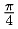# 8.5 Parallel Monte Carlo Computation

## 8.5 Parallel Monte Carlo Computation

One of the types of computation that is easiest to parallelize is the Monte Carlo family of algorithms. In such computations, a random number generator is used to create a number of independent trials. Statistics done with the outcomes of the trials provide a solution to the problem.

We illustrate this technique with another computation of the value of π. If we select points at random in the unit square [0, 1] [0, 1] and compute the percentage of them that lies inside the quarter circle of radius 1, then we will be approximating. (See  for a more detailed discussion together with an approach that does not use a parallel random number generator.) We use the SPRNG parallel random number generator (sprng.cs.fsu.edu). The code is shown in Figure 8.12.` #include "mpi.h" #include <stdio.h> #define SIMPLE_SPRNG        /* simple interface  */ #define USE_MPI             /* use MPI           */ #include "sprng.h"          /* SPRNG header file */ #define BATCHSIZE 1000000 int main( int argc, char *argv[] ) {     int i, j, numin = 0, totalin, total, numbatches, rank, numprocs;     double x, y, approx, pi = 3.141592653589793238462643;     MPI_Init( &argc, &argv );     MPI_Comm_size( MPI_COMM_WORLD, &numprocs );     MPI_Comm_rank( MPI_COMM_WORLD, &rank );     if ( rank == 0 ) {         numbatches = atoi( argv );     }     MPI_Bcast( &numbatches, 1, MPI_INT, 0, MPI_COMM_WORLD );     for ( i = 0; i < numbatches; i++ ) {         for ( j = 0; j < BATCHSIZE; j++) {             x = sprng( ); y = sprng( );             if ( x * x + y * y < 1.0 )                 numin++;         }         MPI_Reduce( &numin, &totalin, 1, MPI_INT, MPI_SUM, 0,                     MPI_COMM_WORLD );         if ( rank == 0 ) {             total = BATCHSIZE * ( i + 1 ) * numprocs;             approx = 4.0 * ( (double) totalin / total );             printf( "pi = %.16f; error = %.16f, points = %d\n",                     approx, pi - approx, total );         }     }     MPI_Finalize( );     return 0; } `Figure 8.12: — Computing π using the Monte Carlo method.

The defaults in SPRNG make it extremely easy to use. Calls to the sprng function return a random number between 0.0 and 1.0, and the stream of random numbers on the different processes is independent. We control the grain size of the parallelism by the constant BATCHSIZE, which determines how much computation is done before the processes communicate. Here a million points are generated, tested, and counted before we collect the results to print them. We use MPI_Bcast to distribute the command-line argument specifying the number of batches, and we use MPI_Reduce to collect at the end of each batch the number of points that fell inside the quarter circle, so that we can print the increasingly accurate approximations to π.Beowulf Cluster Computing With Linux 2003
ISBN: N/A
EAN: N/A
Year: 2005
Pages: 198